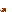# Nominal and real interest rates

Nominal interest rates determine the amount that will be added to the principal of a loan or saving instrument as a cost or return. They are often expressed as a percentage, refer to a specified period of time and are applied to the borrowed or deposited amount. The nominal interest rate can be set in a contract in several different ways (see the relevant section of the Bank Customer Portal).

For example, when home buyers take out a mortgage loan, they must pay the bank the nominal interest rate stipulated in the contract. In other words, if the interest rate on a loan is 3% per annum, the bank will receive interest of €3 for every €100 lent over one year. In the case of deposits, savers will receive the nominal interest rate (the return) for keeping their money at the bank. Bond and other debt instrument holders also receive remuneration in the form of interest or coupons.

The real interest rate is defined as the nominal interest rate minus inflation. By subtracting inflation from the nominal interest rate we can calculate the real cost of a loan and the real return on savings, allowing borrowers and savers to calculate not only the remuneration of their loans or savings in euro (nominal payments), but also the consumer goods they could buy with that remuneration, and thus find out how their purchasing power is affected: Real interest rate = nominal interest rate – inflation

Therefore, the real interest rate varies depending on the nominal interest rate and the rate of inflation.

Let’s look at the difference between nominal and real interest rates through an example. If a saver deposits €100 in a current account at a nominal interest rate of 5%, at the end of the year the balance will be €105. If that same year inflation is 2%, the saver would need €102 to be able to buy the same goods and services that used to cost €100. The real interest rate (i.e. the return on that current account in terms of the consumption basket) is 3%, calculated as the difference between the nominal interest rate (5%) and inflation (2%).